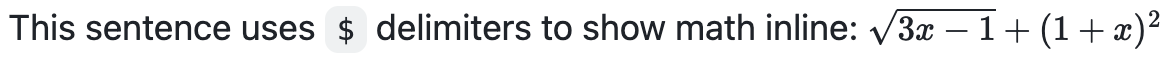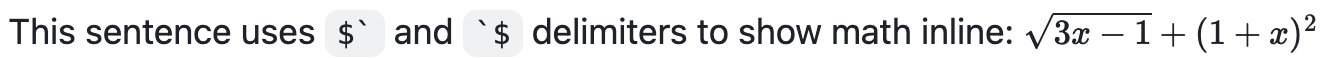# 编写数学表达式

## 关于编写数学表达式

GitHub 的数学呈现功能使用 MathJax；真是一种基于 JavaScript 的开源显示引擎。 MathJax 支持广泛的 LaTeX 宏，以及几个有用的辅助功能扩展。 有关详细信息，请参阅 MathJax 文档MathJax 辅助功能扩展文档

## 编写内联表达式This sentence uses $\ and \$ delimiters to show math inline:  $\sqrt{3x-1}+(1+x)^2$## 将表达式编写为块

**The Cauchy-Schwarz Inequality**

math
\left( \sum_{k=1}^n a_k b_k \right)^2 \leq \left( \sum_{k=1}^n a_k^2 \right) \left( \sum_{k=1}^n b_k^2 \right)



## 在数学表达式所在的行中和数学表达式内编写美元符号

This expression uses \$ to display a dollar sign:$\sqrt{\$4}$• 在数学表达式之外，但在同一行上，在显式 $ 两边使用 span 标记。 To split <span>$</span>100 in half, we calculate $100/2$
`